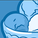Announcements
MAINTENANCE ALERT: Dec. 12th starting 9 AM CET. Community will be read-only. GET DETAILS
cancel
Showing results for
Did you mean:Partner - Contributor II

## Set analysis and Value List

Hi,

Here are my data :

 Product Year Month Quantity Potatoes 2017 1 8 Potatoes 2017 3 13 Apples 2017 1 4 Apples 2017 2 4 Potatoes 2018 1 9 Potatoes 2018 3 6 Apples 2018 1 1 Apples 2018 2 3 Potatoes 2019 1 10 Potatoes 2019 3 15 Apples 2019 1 2 Apples 2019 2 5

I want to create a table that looks like this (based on the selection of a month / year)

 2019 2018 2 YTM 2 YTM Potatoes 0 9 0 9 Apples 5 7 3 4

If I create a table with 4 columns (and without ValueList), it's working, except that the formating sucks

If I try to use the ValueList, one of the value is wrong when there is no data for the current selection :
YTM / Potatoes 2019 displays 9 instead of 10.

See qvw attached.  ValueList.qvw

Does someone has any idea about how to solve that ?

Thanks

Yann

1 Solution

Accepted SolutionsPartner - Contributor II
Author

So, I found the following Workaround :

Do not use ValueList, but create an Island instead
In this case :

Island:
Selection
Year N,
Year N-1];

And replace the formula :
=If(ValueList('Year N', 'Year N-1') = 'Year N',
Sum({\$<Month = {"<= \$(=Only(Month)) "}>}Quantity),
Sum({\$<Month = {"<= \$(=Only(Month)) "}, Year = {"\$(=Only(Year)-1)"}>}Quantity)
)

by :

If(maxstring(TOTAL <Selection>{1} Selection) = 'Year N',
Sum({\$<Month = {"<= \$(=Only(Month)) "}>}Quantity),
Sum({\$<Month = {"<= \$(=Only(Month)) "}, Year = {"\$(=Only(Year)-1)"}>}Quantity)
)Partner - Contributor II
Author

So, I found the following Workaround :

Do not use ValueList, but create an Island instead
In this case :

Island:
Selection
Year N,
Year N-1];

And replace the formula :
=If(ValueList('Year N', 'Year N-1') = 'Year N',
Sum({\$<Month = {"<= \$(=Only(Month)) "}>}Quantity),
Sum({\$<Month = {"<= \$(=Only(Month)) "}, Year = {"\$(=Only(Year)-1)"}>}Quantity)
)

by :

If(maxstring(TOTAL <Selection>{1} Selection) = 'Year N',
Sum({\$<Month = {"<= \$(=Only(Month)) "}>}Quantity),
Sum({\$<Month = {"<= \$(=Only(Month)) "}, Year = {"\$(=Only(Year)-1)"}>}Quantity)
)Community Browser